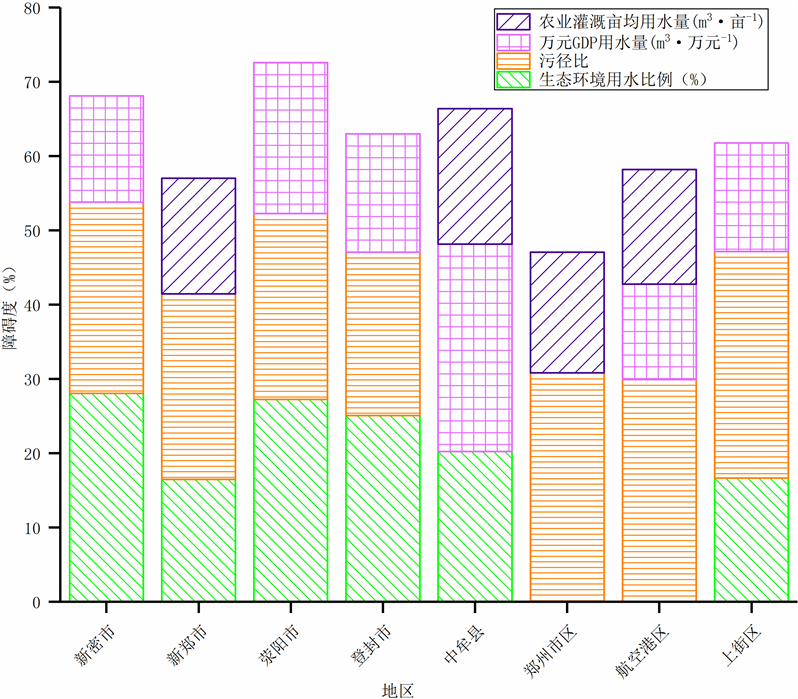# 基于改进灰靶模型的水资源利用效率评价Evaluation of Water Use Efficiency Based on Improved Grey Target Model

DOI: 10.12677/JWRR.2019.84039, PDF, HTML, XML, 下载: 281  浏览: 411  国家自然科学基金支持

Abstract: Water efficiency is a key part of implementing the most stringent water management system, and objective evaluation of it is the prerequisite for water efficiency control. In this paper, the CRITIC weight is introduced into the traditional gray target model for objective weighting, which overcomes the shortcomings of the use of equal weight in the traditional model. An improved gray target model based on CRITIC weight is proposed. At the same time, the obstacle factor diagnosis model is used to analyze the main obstacles affecting water use efficiency. Taking the water efficiency evaluation of various districts in Zhengzhou in 2017 as an example, the evaluation results of the two methods are in good agreement compared with the TOPSIS method, indicating that the improved gray target model has certain application in the evaluation of water use efficiency. Through the analysis of the obstacle degree model, the ecological environment water use index is the main factor hindering the improvement of water use efficiency in Zhengzhou City.

1. 引言

2. 区域概况及评价指标体系

2.1. 研究区域概况

2.2. 评价指标体系及数据来源Table 1. Zhengzhou City water resources utilization efficiency evaluation index system and index weight value

3. 研究方法

3.1. 改进的CRITIC赋权法

CRITIC赋权法由Diakoulaki提出的一种新型客观赋权方法，以指标特征的对比强度和冲突性来综合反映指标的客观权重，它不仅考虑指标内部的变异程度，而且还考虑了指标之间的冲突性，充分考虑了指标的内部和外部特性，赋权结果更为客观、合理。传统的CRITIC赋权法存在下列两方面的不足：第一，带量纲的标准差无法反映出指标内部的对比强度大小；第二，出现负相关时，用相关系数反映指标冲突性显然不合理，参考王瑛等  的研究成果，利用变异系数和带绝对值的Pearson相关系数对传统CRITIC赋权法进行改进，则改进后的赋权公式为：

${C}_{j}={\delta }_{j}\underset{i=1}{\overset{n}{\sum }}\left(1-|{r}_{ij}|\right),j=1,2,\cdots ,n$ (1)

${w}_{j}={C}_{j}/\underset{i=1}{\overset{n}{\sum }}{C}_{j},j=1,2,\cdots ,n$ (2)

3.2. 改进的灰靶模型

$\gamma \left[{x}_{0}\left({u}_{j}\right),{x}_{i}\left({u}_{j}\right)\right]=\frac{\underset{i}{\mathrm{min}}\underset{j}{\mathrm{min}}{\Delta }_{0i}\left({u}_{j}\right)+\rho \underset{i}{\mathrm{max}}\underset{j}{\mathrm{max}}{\Delta }_{0i}\left({u}_{j}\right)}{{\Delta }_{0i}\left({u}_{j}\right)+\rho \underset{i}{\mathrm{max}}\underset{j}{\mathrm{max}}{\Delta }_{0i}\left({u}_{j}\right)}=\frac{0.477}{{\Delta }_{0i}\left({u}_{j}\right)+0.477}$ (3)

$\phi \left({x}_{0},{x}_{i}\right)=\underset{j=1}{\overset{n}{\sum }}{w}_{j}\gamma \left[{x}_{0}\left({u}_{j}\right),{x}_{i}\left({u}_{j}\right)\right]$ (4)

3.3. 水资源利用效率障碍因子诊断模型

${M}_{j}={I}_{j}\cdot {w}_{j}/\left(\underset{j=1}{\overset{n}{\sum }}{I}_{j}\cdot {w}_{j}\right)$ (5)

4. 郑州市水资源利用效率评价

4.1. 郑州市水资源利用效率评价过程Table 2. Actual value of indicator data and gray target transformation resultTable 3. Grey relation difference information space and bull's-eye coefficient, bull's-eye valueTable 4. Results of classification criteria by natural breakpoint method

4.2. 评价结果Table 5. Evaluation results of water resources utilization efficiency in Zhengzhou CityFigure 1. Main obstacle factors and obstacles of water resources utilization efficiency in Zhengzhou City

5. 结论

  李红新. 辽宁省用水效率的时空变化分析及影响因素研究[D]: [硕士学位论文]. 大连: 辽宁师范大学, 2008. LI Hongxin. Analysis on spatial-temporal changes of water use efficiency and influencing factors in Liaoning Province. Master’s Degree Thesis. Dalian: Liaoning Normal University, 2008. (in Chinese)  张旭腾, 于静洁. 基于非期望输出DEA模型的各省用水效率评价[J]. 水资源研究, 2017, 6(2): 138-147. ZHANG Xuteng, YU Jingjie. Water use efficiency assessment of Chinese provinces based on a data envelopment analysis model with undesirable outputs. Journal of Water Resources Research, 2017, 6(2): 138-147. (in Chinese)  朱燕飞, 陈智和, 金远征. 金华市水资源利用效率评价研究[J]. 人民长江, 2016, 47(21): 43-47. ZHU Yanfei, CHEN Zhihe and JIN Yuanzheng. Evaluation on utilization efficiency of water resources in Jinhua City. Yangtze River, 2016, 47(21): 43-47. (in Chinese)  王晓琳, 吴凯, 许怡. 基于物元可拓模型的区域用水效率控制综合评价[J]. 水电能源科学, 2017, 35(3): 30-34. WANG Xiaolin, WU Kai and XU Yi. Comprehensive evaluation of regional water use efficiency control based on Mat-ter-element model. Water Resources and Power, 2017, 35(3): 30-34. (in Chinese)  孟令爽, 唐德善, 史毅超. 基于主成分分析法的用水效率评价[J]. 人民长江, 2018, 49(5): 36-40. MENG Lingshuang, TANG Deshan and SHI Yichao. Evaluation on water use efficiency based on principal components analysis. Yangtze River, 2018, 49(5): 36-40. (in Chinese)  段长桂, 董增川, 管西柯, 等. 基于迭代思想的山东省水资源利用效率评价[J]. 人民黄河, 2017, 39(12): 62-66. DUAN Changgui, DONG Zengchuan, GUAN Xike, et al. Evolution for water use efficiency of Shandong Province based on circulation correction model. Yellow River, 2017, 39(12): 62-66. (in Chinese)  余兴奎, 何士华, 高飞. 云南省水资源利用效率评价[J]. 中国农村水利水电, 2012(3): 87-90. YU Xingkui, HE Shihua and GAO Fei. Evaluation of water use efficiency in Yunnan Province. China Rural Water and Hydropower, 2012(3): 87-90. (in Chinese)  朱兆珍, 梁中. 我国省域水资源利用效率评价研究[J]. 河海大学学报(哲学社会科学版), 2015, 17(3): 72-78+92. ZHU Zhaozhen, LIANG Zhong. Research into evaluation of efficiency of provincial water resource use in China. Journal of Hohai University (Philosophy and Social Sciences), 2015, 17(3): 72-78+92. (in Chinese)  李恩宽, 蔡大应, 赵焱, 等. 基于混合蛙跳算法投影寻踪模型的黄河流域用水效率评价[J]. 中国农村水利水电, 2016(5): 88-91. LI Enkuan, CAI Daying, ZHAO Yan, et al. Evaluation of water use efficiency using the projection pursuit model based on the Shuffled Frog Leaping Algorithm in the Yellow River basin. China Rural Water and Hydropower, 2016(5): 88-91. (in Chi-nese)  管新建, 秦海东, 孟钰. 基于CRITIC-TOPSIS-灰色关联度的淮河流域水资源利用效率评估[J]. 节水灌溉, 2018, 279(11): 73-76+80. GUAN Xinjian, QIN Haidong and MENG Yu. Water use efficiency evaluation of Huai River basin based on CRITIC-TOPSIS-Gray relation degree. Water Saving Irrigation, 2018, 279(11): 73-76+80. (in Chinese)  邓聚龙. 灰理论基础[M]. 武汉: 华中科技大学出版社, 2002: 171-201. DENG Julong. Foundation of Grey Theory. Wuhan: Huazhong University of Science and Technology Press, 2002: 171-201. (in Chinese)  侯玉乐, 李钢, 渠俊峰, 等. 基于改进灰靶模型的土地生态安全评价——以江苏省徐州市为例[J]. 水土保持研究, 2017, 24(1): 285-290. HOU Yule, LI Gang, QU Junfeng, et al. Evaluation of land eco-security based on improved Grey Target Model—A case of Xuzhou City, Jiangsu Province. Research of Soil and Water Conservation, 2017, 24(1): 285-290. (in Chinese)  文博, 刘友兆, 夏敏, 等. 基于灰靶模型的宜兴市农村居民点布局适宜性评价及分类调控研究[J]. 地域研究与开发, 2016, 35(5): 153-157+169. WEN Bo, LIU Youzhao, XIA Min, et al. Suitability evaluation and regulation of rural residential land in Yixing City based on the Grey Target Model. Areal Research and Development, 2016, 35(5): 153-157+169. (in Chinese)  熊鸿斌, 周凌燕. 基于PSR-灰靶模型的巢湖环湖防洪治理工程生态环境影响评价研究[J]. 长江流域资源与环境, 2018, 27(9): 1977-1987. XIONG Hongbin, ZHOU Lingyan. Using PSR-Grey Target Model to assess ecological and environmental impact of flood regulation project at riparian zone around Lake Chaohu. Resources and Environment in the Yangtze Basin, 2018, 27(9): 1977-1987. (in Chinese)  杨丽英, 许新宜, 贾香香. 水资源效率评价指标体系探讨[J]. 北京师范大学学报(自然科学版), 2009, 45(5): 642-646. YANG Liying, XU Xinyi and JIA Xiangxiang. Water use efficiency evaluating index system. Journal of Beijing Normal University (Natural Science), 2009, 45(5): 642-646. (in Chinese)  王瑛, 姜芸芸. 基于改进CRITIC赋权法和模糊优选法的大气质量评价[J]. 统计与决策, 2017(17): 83-87. WANG Ying, JIANG Yunyun. Air quality assessment based on improved CRITIC weighting method and fuzzy optimization method. Statistics & Decision, 2017(17): 83-87. (in Chinese)